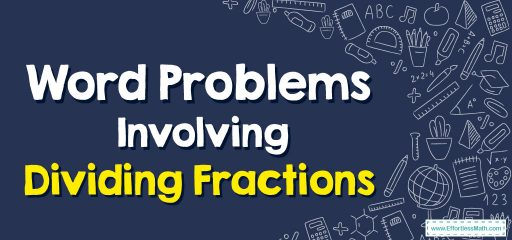# How to Solve Word Problems Involving Dividing Fractions

This article provides a step-by-step guide for solving math word problems that involve the division of fractions.## A step-by-step guide toword problems involving dividing fractions

The word problems of fractions are made of a few sentences involving a real-life scenario and you must use the mathematical calculation of fraction formulas to solve a problem.

Here are easy steps to solve word problems involving dividing fractions:
Step 1: Use information and keywords to identify the problems.
Step 2: Invert the second fraction and multiply instead of dividing.
Step 3: Multiply the 2 numerators. Multiply the 2 denominators. If the problem contains mixed numbers, you must convert them to improper fractions first.
Step 4: Simplify the fraction if possible.

### Word Problems Involving Dividing Fractions – Example 1

Karolina uses $$\frac{6}{8}$$ of a jar of cherry jam to make eight muffins. What fraction of the jar of cherry jam does each muffin contain?

Solution:
Step 1: Divide the total amount of a used cherry jam by the number of muffins, $$\frac{6}{8}÷8$$.
Step 2: Write 8 as an improper fraction, $$\frac{6}{8}÷\frac{8}{1}$$.
Step 3: Invert the second fraction and multiply instead of dividing.
$$\frac{6}{8}×\frac{1}{8}=\frac{6×1}{8×8}=\frac{6}{64}$$
Step 4: Simplify the product. $$\frac{6}{64}=\frac{3}{32}$$

### Word Problems Involving Dividing Fractions – Example 2

Alice uses $$\frac{4}{6}$$ of a roll of wrapping paper to wrap five presents. What fraction of the roll wrapping paper does each present use?

Solution:
Step 1: Divide the total amount of a roll of wrapping paper by the number of presents. $$\frac{4}{6}÷5$$
Step 2: Write 5 as an improper fraction. $$\frac{4}{6}÷\frac{5}{1}$$
Step 3: Invert the second fraction and multiply instead of dividing.
$$\frac{4}{6}×\frac{1}{5}=\frac{4×1}{6×5}=\frac{4}{30}$$
Step 4: Simplify the product. $$\frac{4}{30}=\frac{2}{15}$$

### What people say about "How to Solve Word Problems Involving Dividing Fractions - Effortless Math: We Help Students Learn to LOVE Mathematics"?

No one replied yet.

X
30% OFF

Limited time only!

Save Over 30%

SAVE $5 It was$16.99 now it is \$11.99